# R S Aggarwal Solutions for Class 10 Maths Exercise 18D Chapter 18 Mean, Median, Mode of Grouped Data, Cumulative Frequency Graph and Ogive

In this section, students will get answers for all the questions enlisted under exercise 18D. R S Aggarwal Solutions for this exercise mentioned below are solved by subject experts using step by step problem solving approach. Students can download Class 10 Maths Chapter 18 R S Aggarwal Solutions and score well in exams.

## Download PDF of R S Aggarwal Solutions for Class 10 Maths Chapter 18 Mean, Median, Mode of Grouped Data, Cumulative Frequency Graph and Ogive Exercise 18D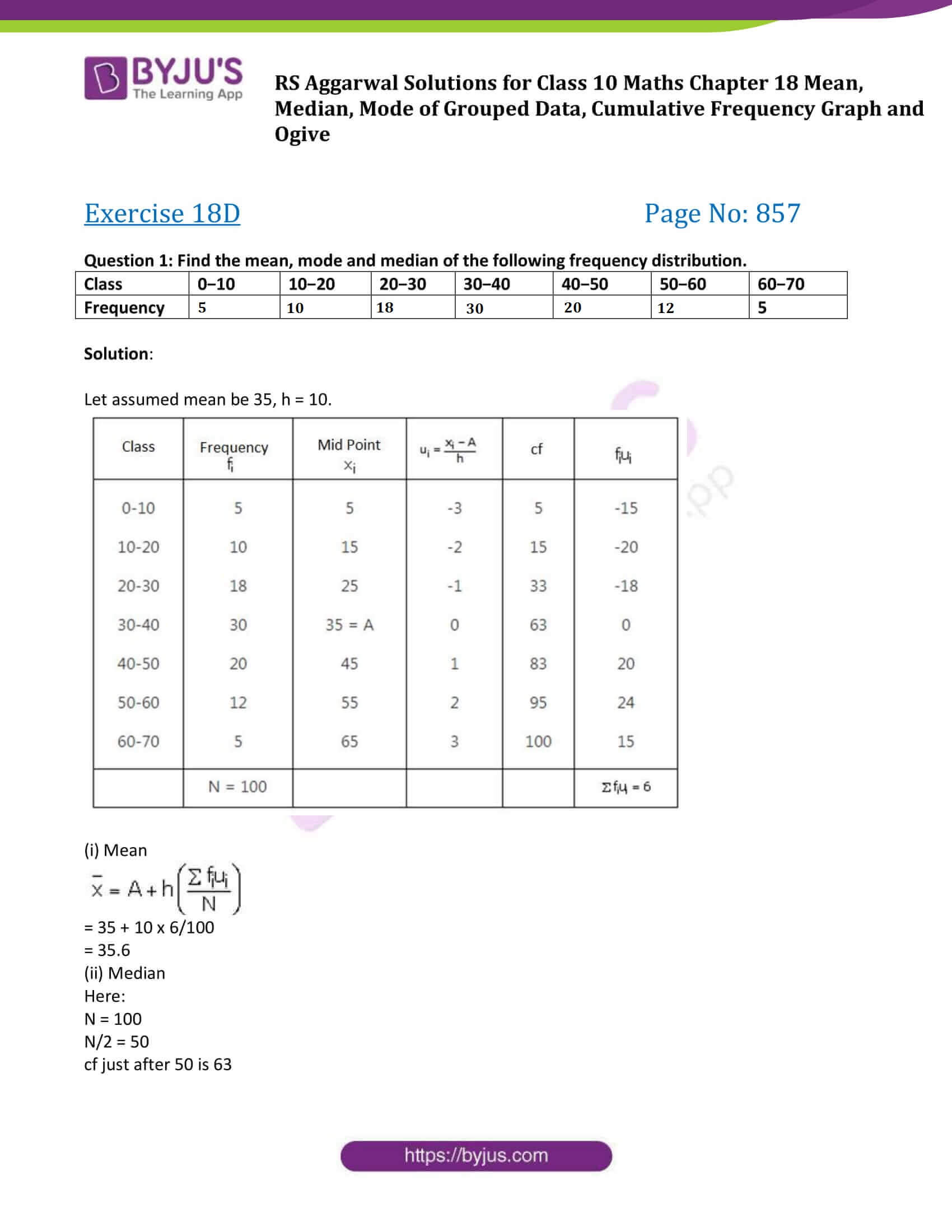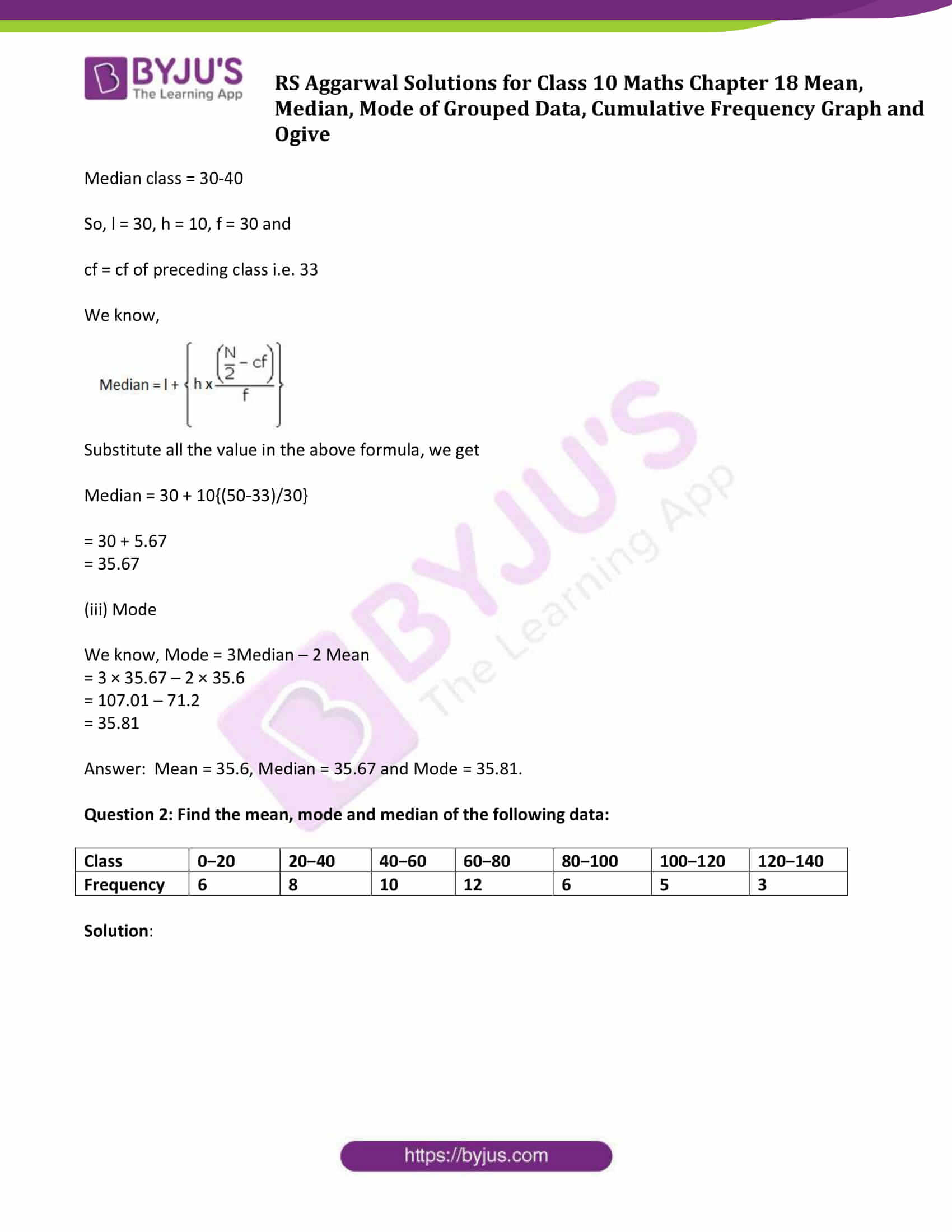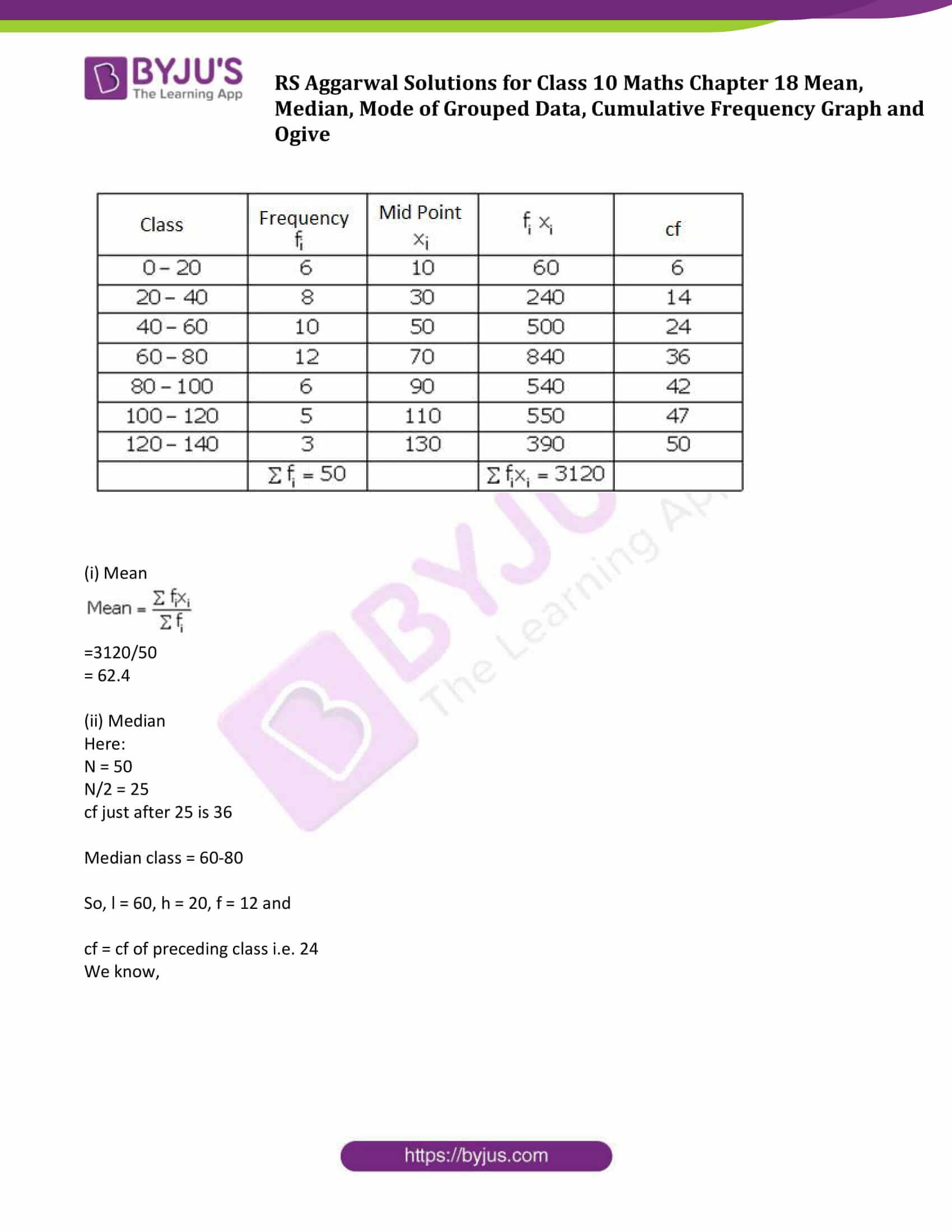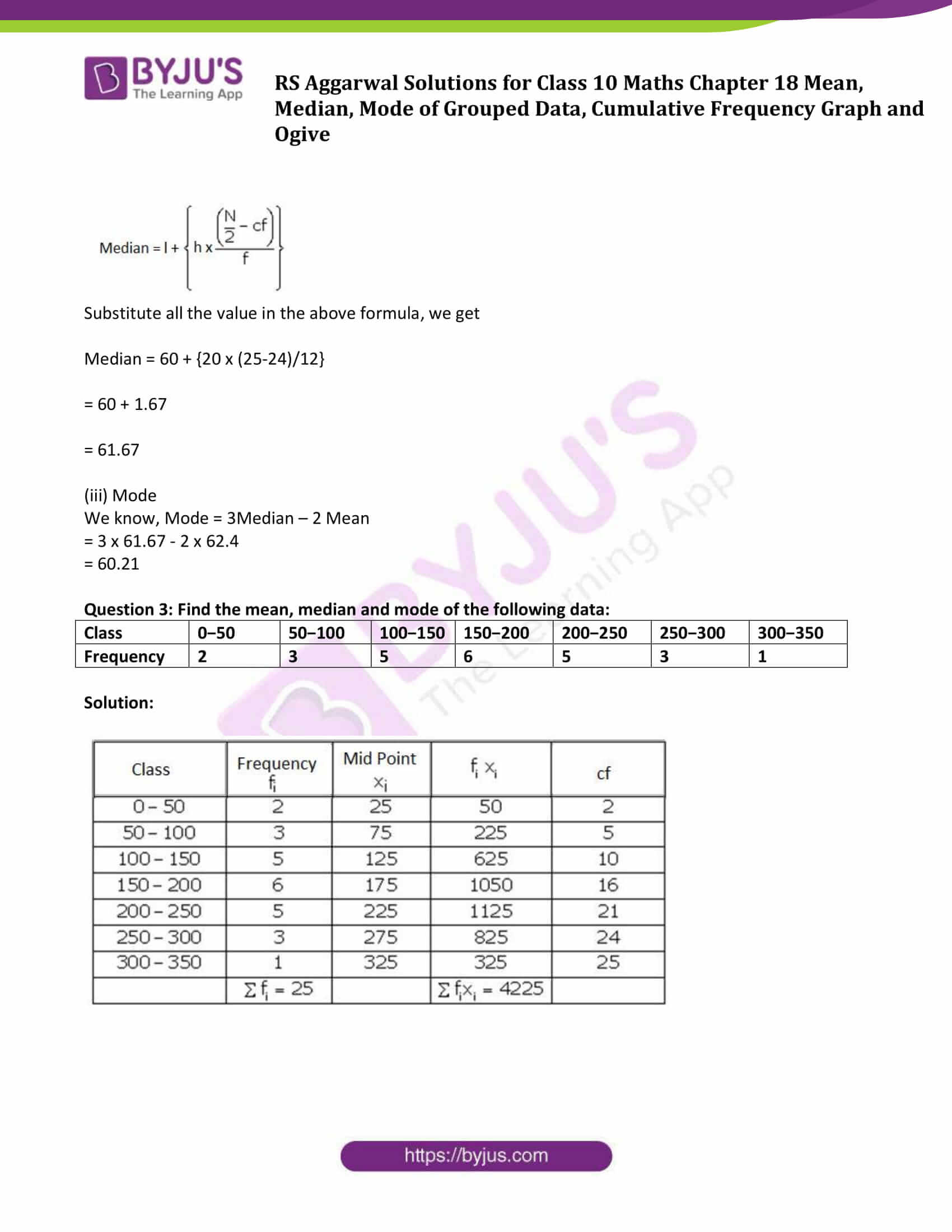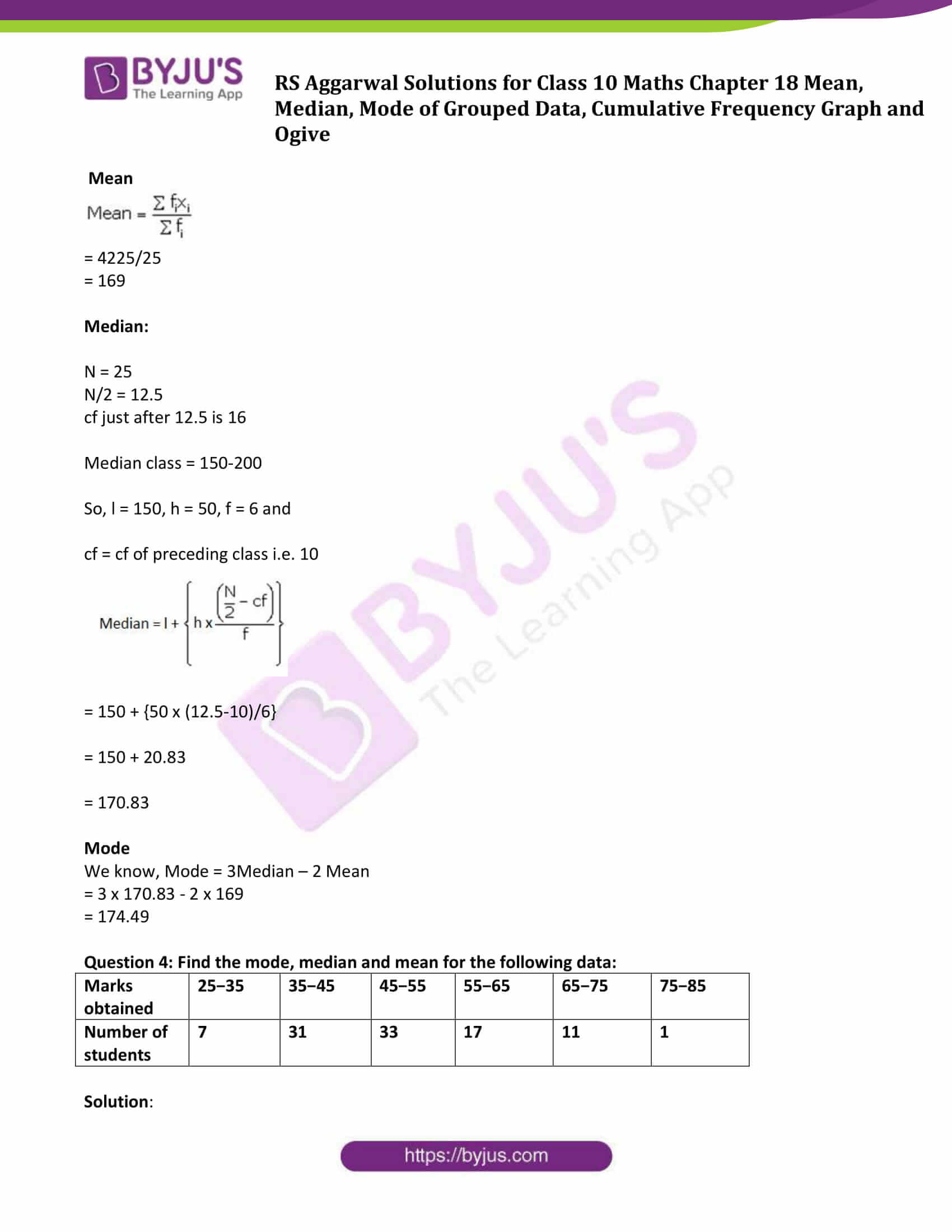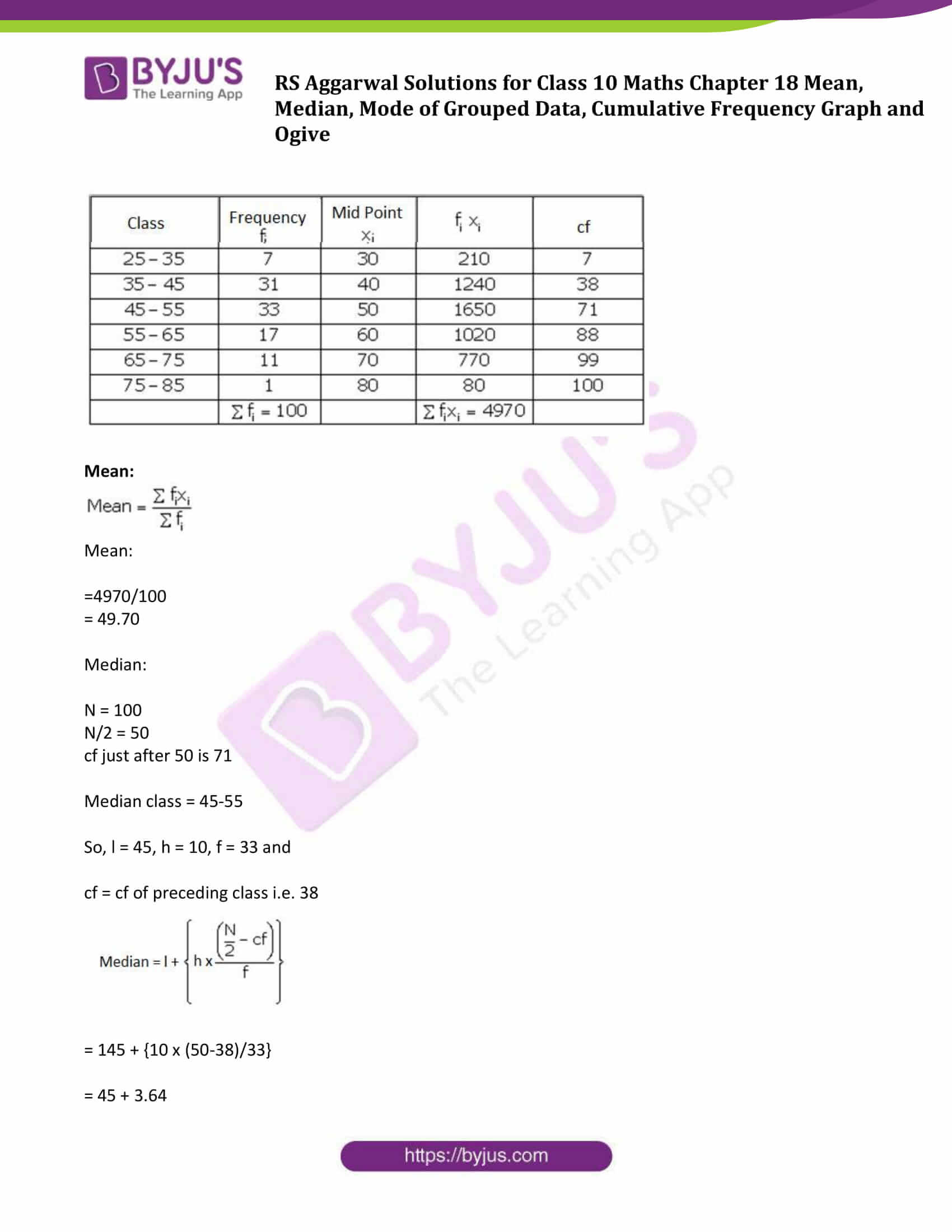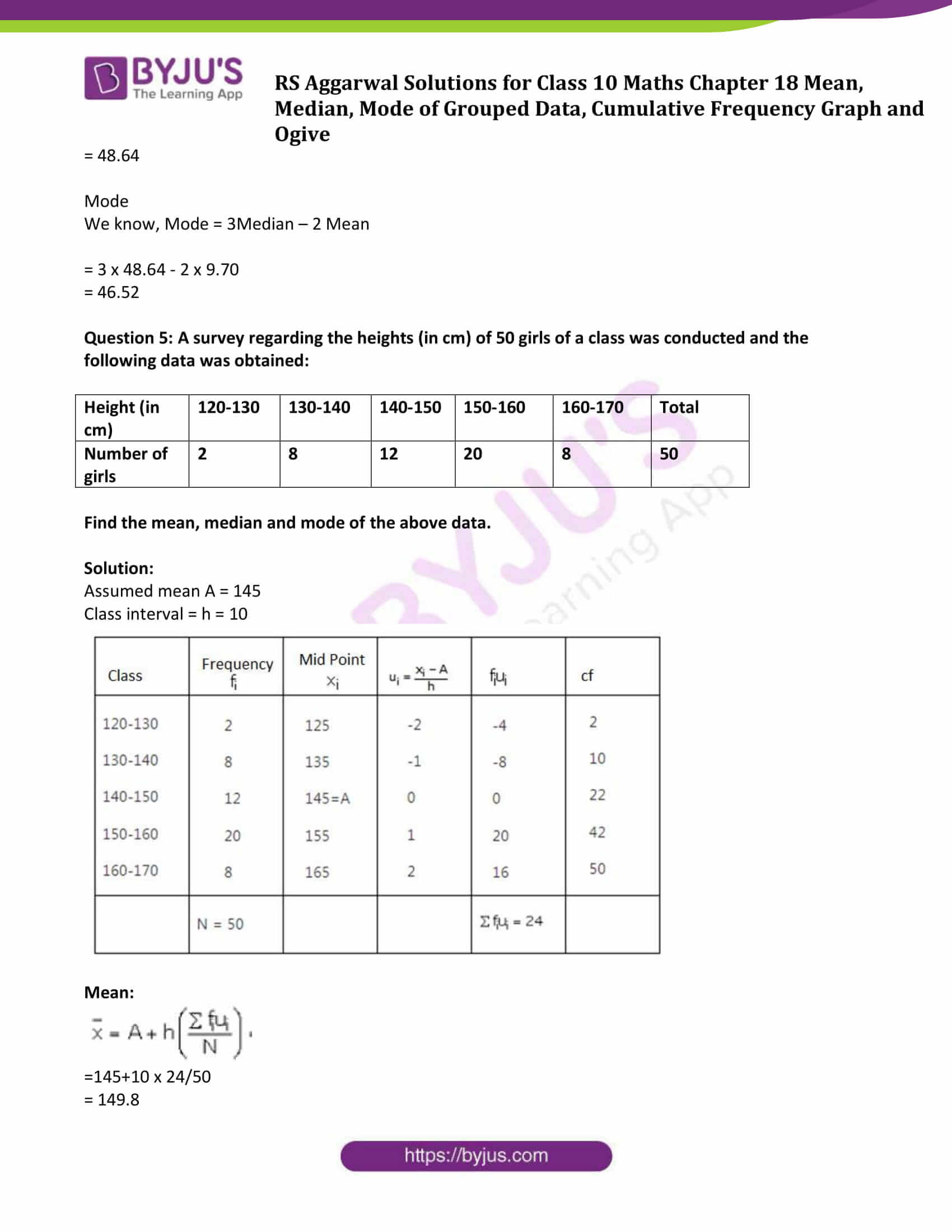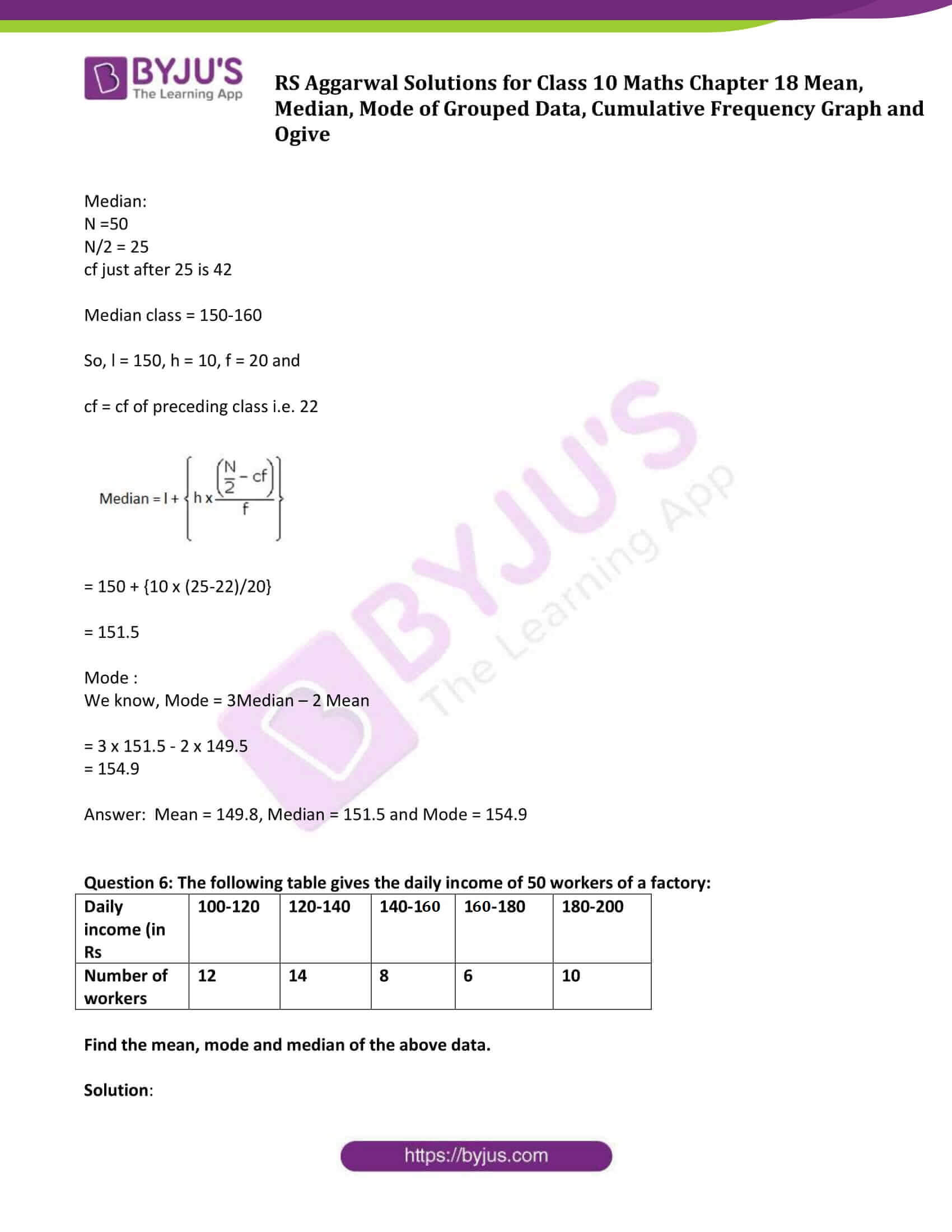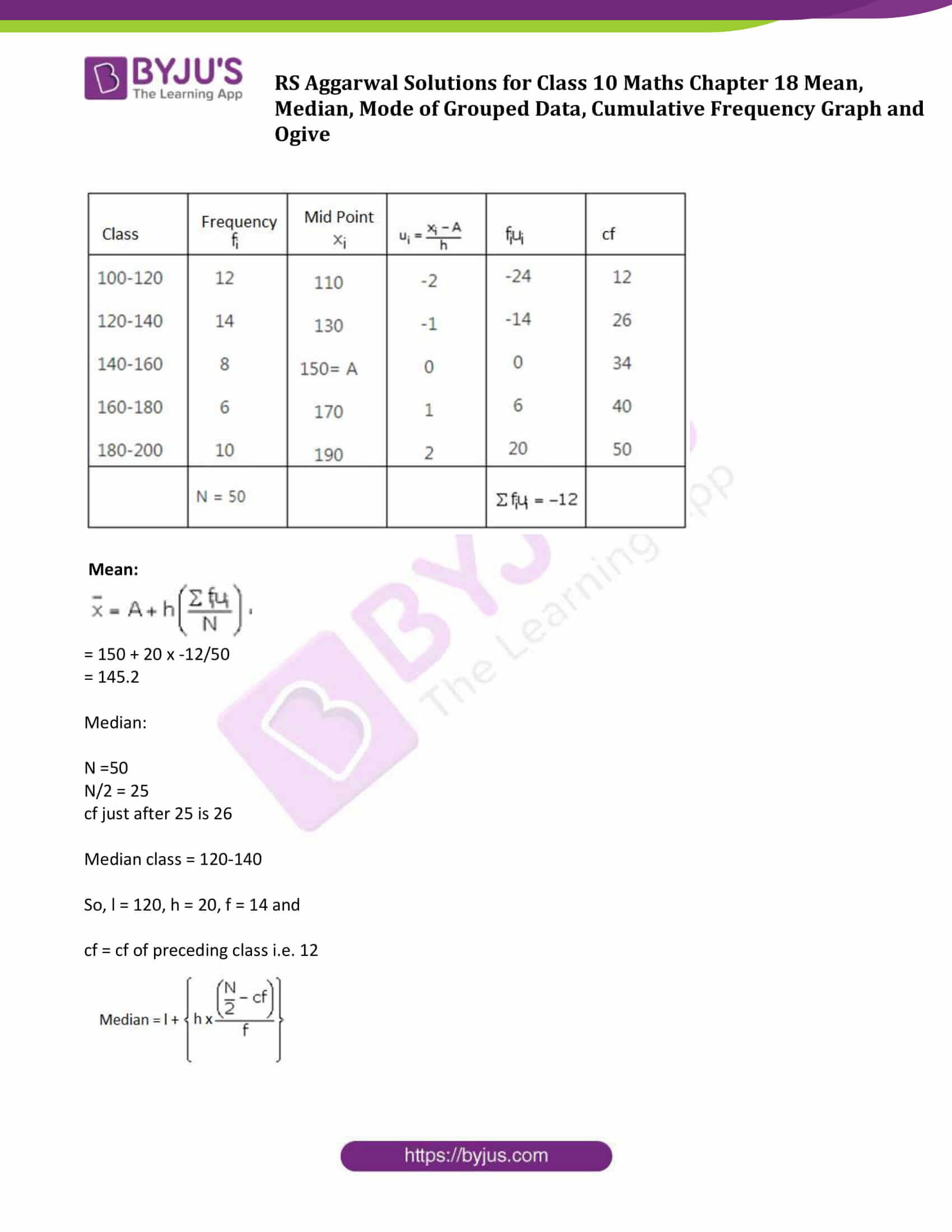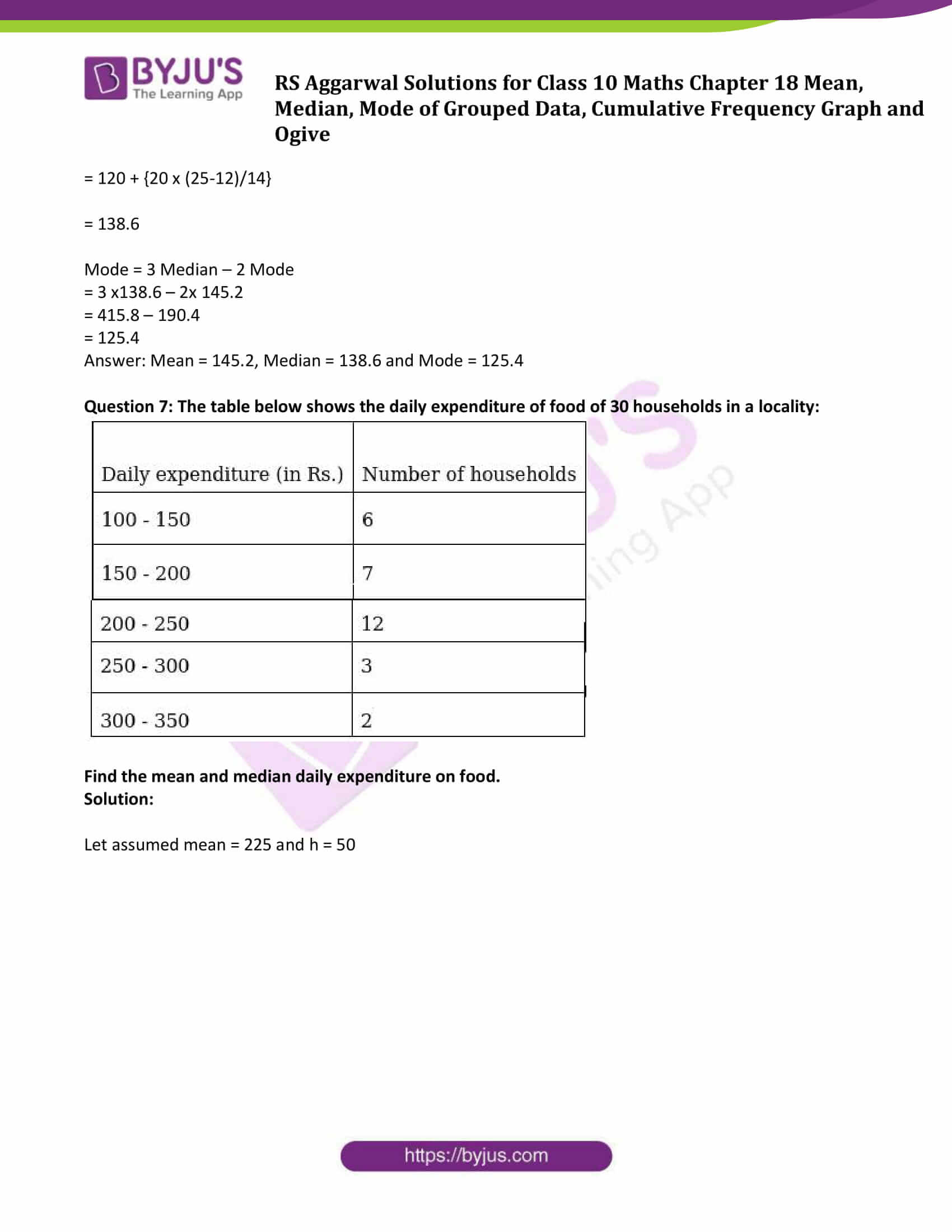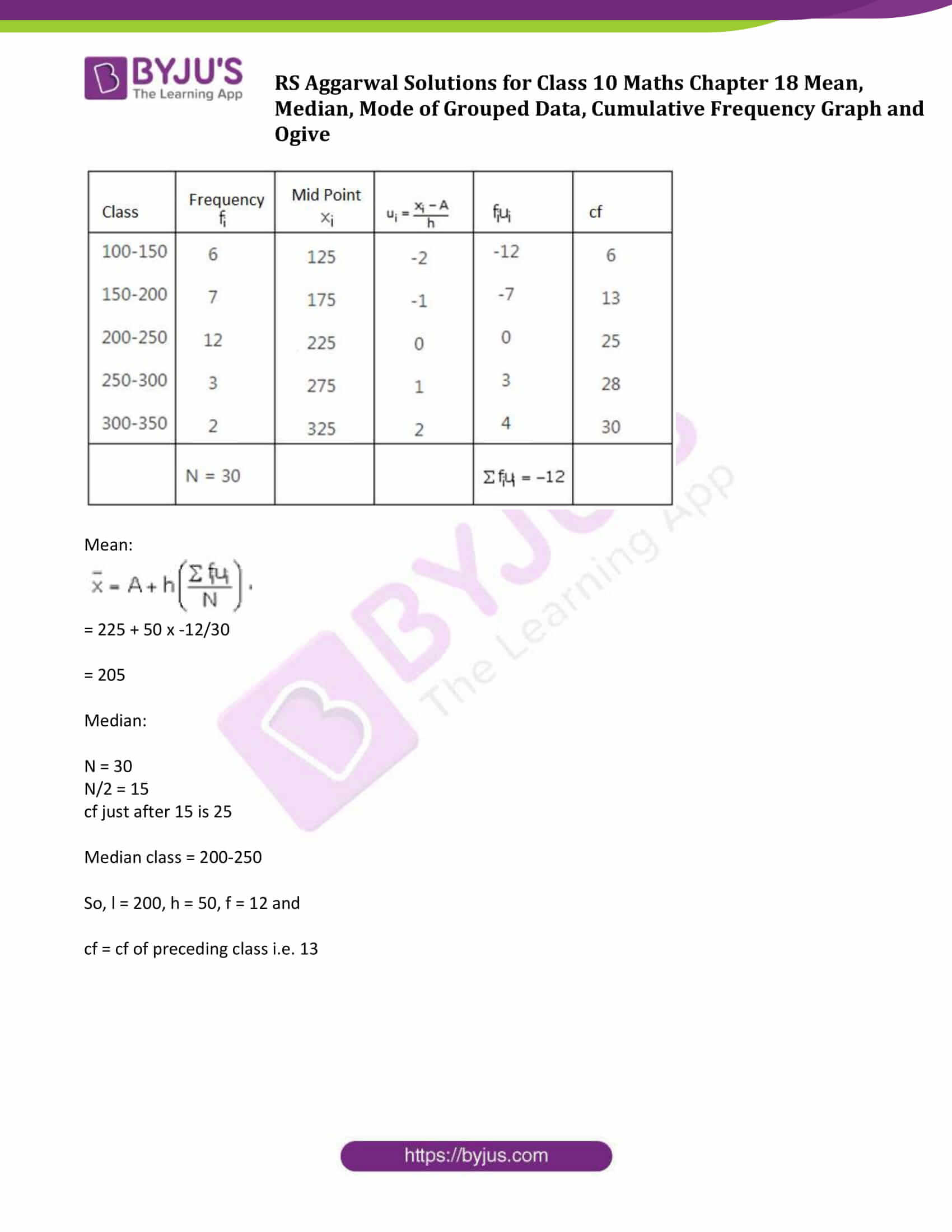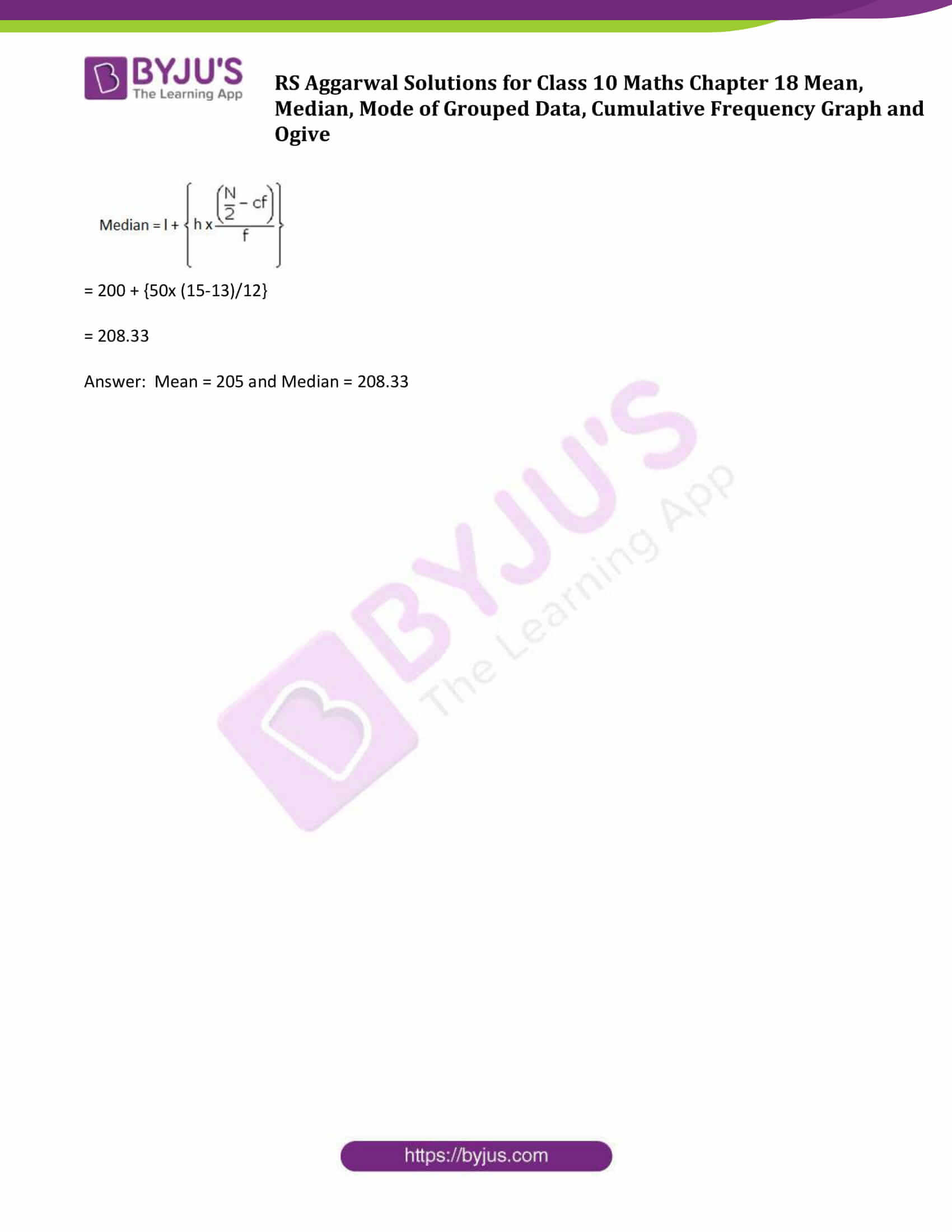## Access other exercise solutions of Class 10 Maths Chapter 18 Mean, Median, Mode of Grouped Data, Cumulative Frequency Graph and Ogive

Exercise 18 A Solutions

Exercise 18 B Solutions

Exercise 18 C Solutions

Exercise 18 E Solutions

## Exercise 18D

Question 1: Find the mean, mode and median of the following frequency distribution.

 Class 0−10 10−20 20−30 30−40 40−50 50−60 60−70 Frequency 5 10 18 30 20 12 5

Solution:

Let assumed mean be 35, h = 10.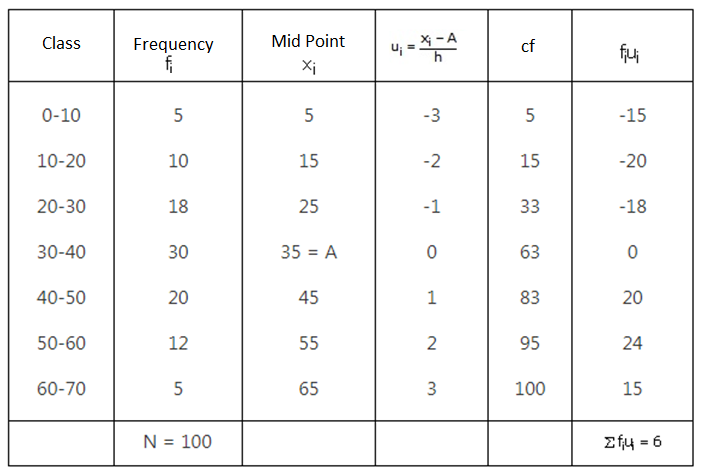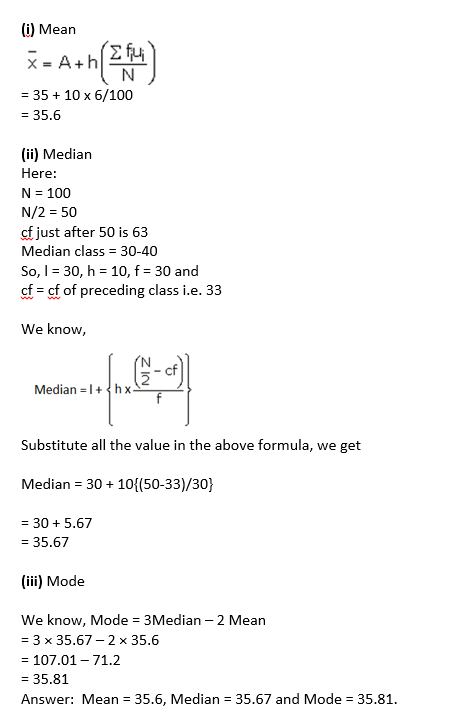Question 2: Find the mean, mode and median of the following data:

 Class 0−20 20−40 40−60 60−80 80−100 100−120 120−140 Frequency 6 8 10 12 6 5 3

Solution: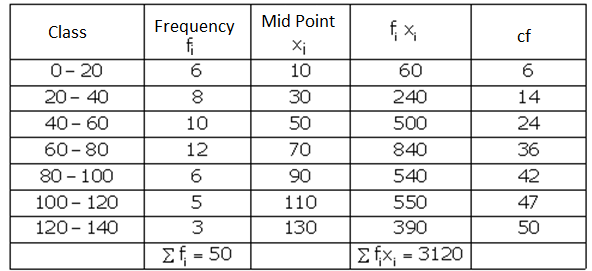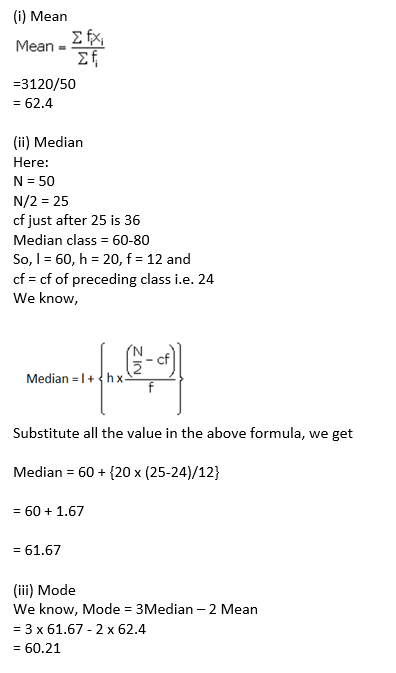Question 3: Find the mean, median and mode of the following data:

 Class 0−50 50−100 100−150 150−200 200−250 250−300 300−350 Frequency 2 3 5 6 5 3 1

Solution: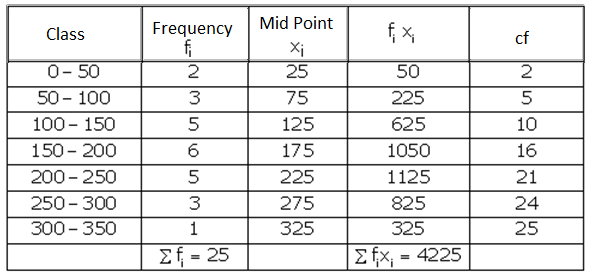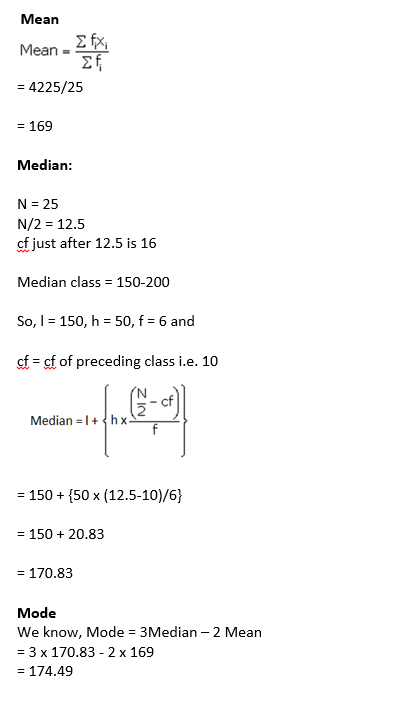Question 4: Find the mode, median and mean for the following data:

 Marks obtained 25−35 35−45 45−55 55−65 65−75 75−85 Number of students 7 31 33 17 11 1

Solution: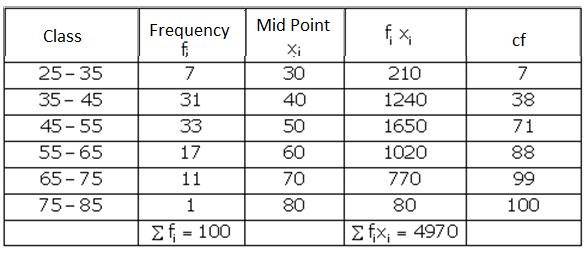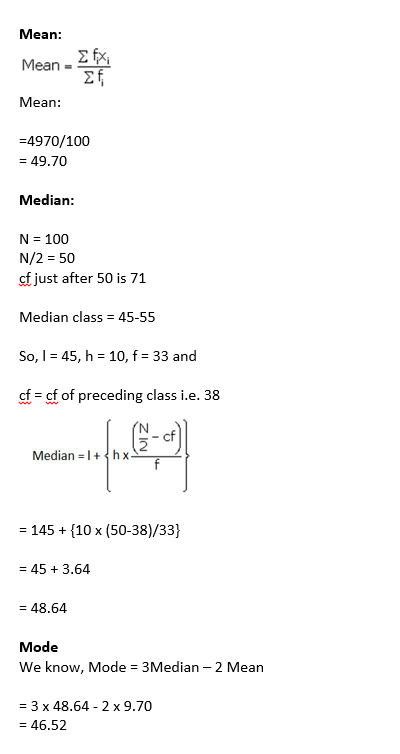Question 5: A survey regarding the heights (in cm) of 50 girls of a class was conducted and the following data was obtained:

 Height (in cm) 120-130 130-140 140-150 150-160 160-170 Total Number of girls 2 8 12 20 8 50

Find the mean, median and mode of the above data.

Solution:

Assumed mean A = 145

Class interval = h = 10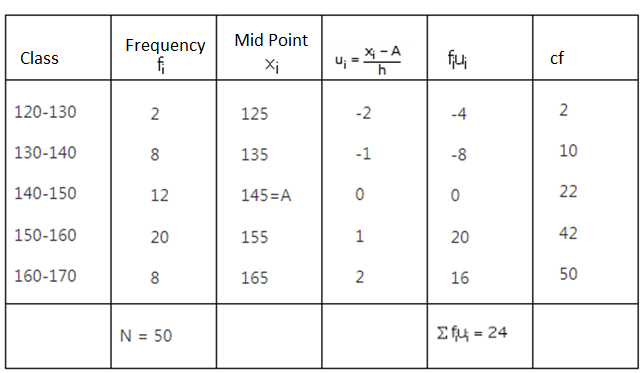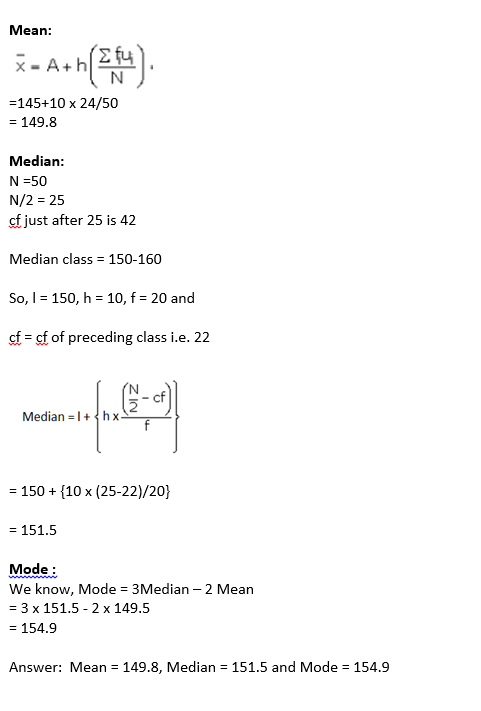Question 6: The following table gives the daily income of 50 workers of a factory:

 Daily income (in Rs 100-120 120-140 140-160 160-180 180-200 Number of workers 12 14 8 6 10

Find the mean, mode and median of the above data.

Solution: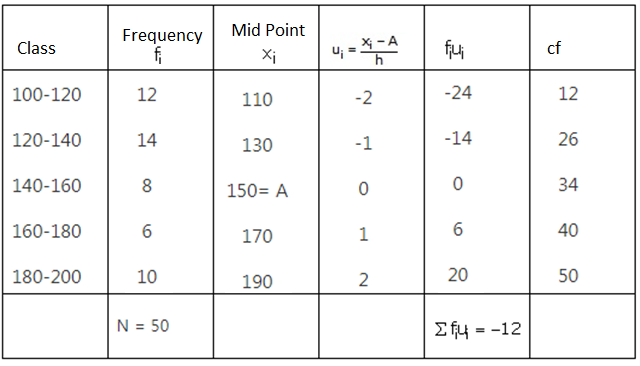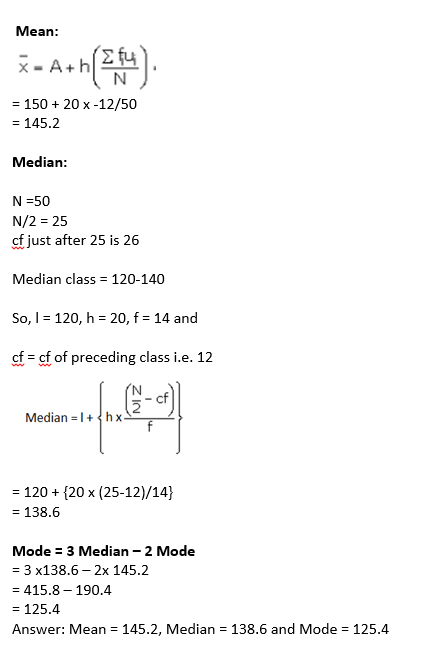Question 7: The table below shows the daily expenditure of food of 30 households in a locality: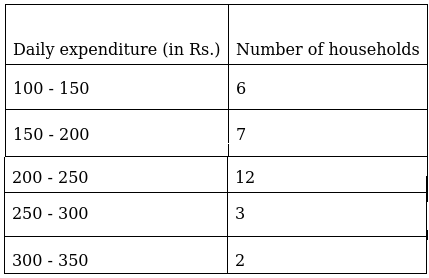Find the mean and median daily expenditure on food.

Solution:

Let assumed mean = 225 and h = 50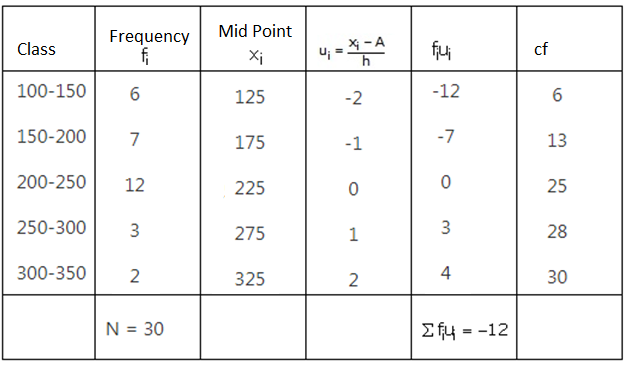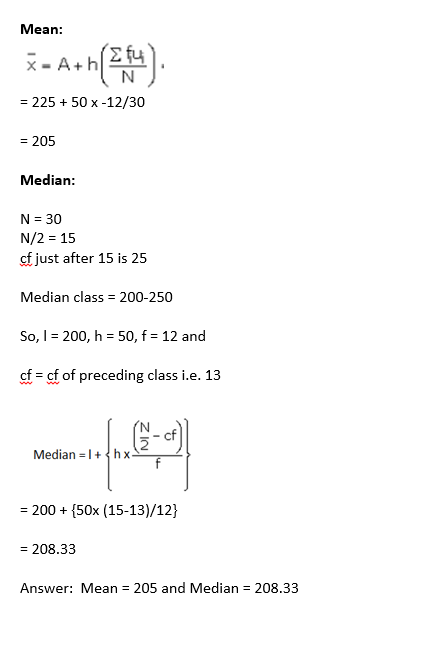Class 10 Maths Chapter 18 Mean, Median, Mode of Grouped Data, Cumulative Frequency Graph and Ogive Exercise 18D is based on Measures of central tendency.

Empirical relationship between the three measures of central tendency:

3 Median = Mode + 2 Mean